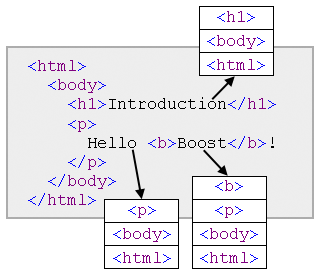#Boost C++ Libraries

...one of the most highly regarded and expertly designed C++ library projects in the world.

# Boost.Flyweight Examples

## Example 1: basic usage

See source code.

Dummy program showing the basic capabilities of `flyweight` explained at the tutorial.

## Example 2: key-value flyweights

See source code.

The program simulates the scenario described at the tutorial section on key-value flyweights: The class `texture` manages some texture rendering data stored in a file whose location is given at construction time. The program handles large quantities of objects of this class by encapsulating them into key-value flyweights keyed by filename. Observe how the execution of the program results in no extra constructions or copies of objects of type `texture` except those absolutely necessary.

## Example 3: flyweights and the composite pattern

See source code.

The composite design pattern revolves about the idea that a tree data structure can be easily constructed and manipulated by defining the tree node type polymorphically so that either is a leaf node or else contains a list of pointers to their child nodes. This way, a tree is the exact same entity as its root node, which allows for very simple recursive tree-handling algorithms. Large composite trees having a high degree of duplication of nodes and subtrees (as for instance those generated when parsing a computer program) are a natural fit for the flyweight idiom: simply turning the node type into a flyweight automatically deals with duplication at the node and subtree level.

The example program parses Lisp-like lists of the form `(a1 ... an)` where each `ai` is a terminal string or a list. The parsed data structure is a composite type defined using Boost.Flyweight in conjunction with the recursive facilities of Boost.Variant. So, given the list

```(= (tan (+ x y))(/ (+ (tan x)(tan y))(- 1 (* (tan x)(tan y)))))
```

the resulting data structure implicitly detects the duplicated occurrences of `+`, `x`, `y`, `tan`, `(tan x)` and `(tan y)`.

## Example 4: formatted text processing

See source code.

A classic example of application of the flyweight pattern is that of a text processor which handles characters with rich formatting information, like font type, size, color and special options (boldness, italics, etc.) Coding the formatting information of each character takes considerable space, but, given the high degree of repetition typical in a document, maintaining formatted characters as flyweight objects drastically reduces memory consumption.

The example program parses, manipulates and stores HTML documents following flyweight-based representation techniques. Given the hierarchical nature of HTML markup, a crude approximation to the formatting options of a given character is just to equate them with the stack of tag contexts to which the character belongs, as the figure shows.Fig. 1: Formatting contexts of characters in an HTML document.

HTML documents are then parsed as arrays of (character, format) pairs, where the format is the tag context as described above. The very high degree of redundancy in formatting information is taken care of by the use of Boost.Flyweight. This character-based representation makes it easy to manipulate the document: transposition and elimination of portions of text are trivial operations. As an example, the program reverses the text occupying the central portion of the document. Saving the result in HTML reduces to traversing the array of formatted characters and emitting opening/closing HTML tags as the context of adjacent characters varies.

For the sake of brevity, the HTML parsing capabilities of this program are coarse: for instance, elements without end-tag (like <BR>), character enconding and HTML entities (e.g. "&copy;" for ©) are not properly handled. Improving the parsing code is left as an exercise to the reader.

## Example 5: flyweight-based memoization

See source code.

Memoization is an optimization technique consisting in caching the results of a computation for later reuse; this can dramatically improve performance when calculating recursive numerical functions, for instance. Key-value flyweights can be used to implement memoization for a numerical function f by modeling a memoized invocation of the function as a value of type `flyweight<key_value<int,compute_f> >`, where `compute_f` is a type that does the computation of f(n) at its `compute_f::compute_f(int)` constructor. For instance, the Fibonacci numbers can be computed with memoization like this:

```typedef flyweight<key_value<int,compute_fibonacci>,no_tracking> fibonacci;

struct compute_fibonacci
{
compute_fibonacci(int n):
result(n==0?0:n==1?1:fibonacci(n-2).get()+fibonacci(n-1).get())
{}

operator int()const{return result;}
int result;
};
```

The `no_tracking` policy is used so that the memoized computations persist for future use throughout the program. The provided program develops this example in full.

## Example 6: performance comparison

See source code.

This program measures the time and space performances of a simple string type against several differently configured `flyweight` instantations as used in a conventional task involving parsing a file and doing some manipulations on the parsed text. Memory consumption is computed by instrumenting the relevant components (the string type itself, flyweight factories, etc.) with custom allocators that keep track of the allocations and deallocations requested. The program has been used to produce the experimental results given at the performance section.

## Example 7: custom factory

See source code.

The example shows how to write and use a custom factory class. This "verbose" factory outputs messages tracing the invocations of its public interface by Boost.Flyweight, so helping the user visualize factory usage patterns.

Revised December 2nd 2008